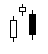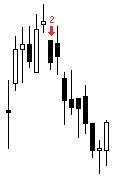# EveningStar

 Description The Evening Star pattern is the converse of the Morning Star pattern. The Evening Star is a top reversal, so bullish trend confirmation is important.   The Evening Star is a three candlestick pattern:Ideally, the first, white bar is has a long white body. The second candlestick gaps up and has a small real body--ideally, no real body (Doji). The third candlestick has a black body that penetrates well into the first day's white real body. If the third candlestick gaps down, an Abandoned Baby pattern (Island Top) emerges. Formula EveningStar(INPUT, Stringency=5, LRRange=10, LRType=3, DojiPercentage=15)=begin   retval = NONUM   targetBar = 0   pnt0 = targetBar   pnt1 = targetBar + 1   pnt2 = targetBar + 2   ppClose = GetPrice(\$1, BATE_CLOSE, pnt2, 0)   ppLow = GetPrice(\$1, BATE_LOW, pnt2, 0)   pOpen = GetPrice(\$1, BATE_OPEN, pnt1, 0)   pClose = GetPrice(\$1, BATE_CLOSE, pnt1, 0)   pLow = GetPrice(\$1, BATE_LOW, pnt1, 0)   cOpen = GetPrice(\$1, BATE_OPEN, targetBar, 0)     if Bullish(\$1, pnt2, LRRange, LRType) == TRUE then begin     if BlackBody(\$1, pnt0) == TRUE then begin       if WhiteBody(\$1, pnt2) == TRUE then begin         if Stringency >= 7 then retval = '7'|clr_red|above|ftiny|arrow|vertical         if pOpen > ppClose then begin           if Stringency >= 6 then retval = '6'|clr_red|above|ftiny|arrow|vertical           if cOpen < pClose then begin             if Stringency >= 5 then retval = '5'|clr_red|above|ftiny|arrow|vertical             if DojiForm(\$1, pnt1, DojiPercentage) then begin               if Stringency >= 4 then retval = '4'|clr_red|above|ftiny|arrow|vertical               if cOpen < pLow then begin                 if Stringency >= 3 then retval = '3'|clr_red|above|ftiny|arrow|vertical                 if LongWhiteForm(\$1, pnt2) then begin                   if Stringency >= 2 then retval = '2'|clr_red|above|ftiny|arrow|vertical                   if LongBlackForm(\$1, pnt1) then begin                     retval = 'Evening Star'|clr_black|above|ftiny|arrow|vertical                   end                 end               end             end           end         end       end     end   end   retval end Parameters INPUT The INPUT directive makes this formula available as a Formula Overlay. INPUT refers to the instrument in the chart.   Stringency The Stringency argument is used by nearly all candlestick overlays. It controls how many tests must pass in the evaluation of an Evening Star. The lower the number, the more strict the interpretation.   The Stringency argument is an overlay parameter. You can adjust it using the Parameters dialog. The default is 5.   LRRange The EveningStar overlay uses the LR() function with either a continuous or quadratic calculation to determine how the market is trending. The LRRange (Linear Regression Range) parameter is the number of points in the Linear Regression calculation. The default is 10.   LRType The LRType parameter has two possible settings: 2 and 3, or continuous linear regression and quadratic linear regression, respectively. The default is 3, or quadratic.   DojiPercentage The DojiPercentage parameter represents a percentage. This parameter serves as the basis for determining whether a candlestick is Doji or near Doji. The smaller the value assigned to DojiPercentage the more stringent the test. The default is 15, or fifteen percent. Return Value The return value is a string plot and varies depending on the value of the Stringency parameter.  The lower the number, the stronger the pattern.   The string plot for a fully qualified Evening Star pattern is "Evening Star" rendered in black above the candlestick. ExamplesComments NA